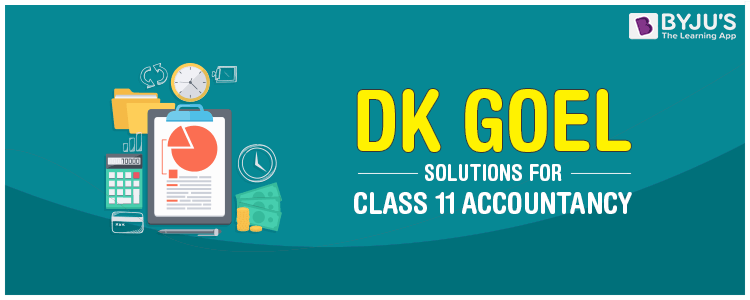# DK Goel Solutions for Class 11 AccountancyStudents first come across Accountancy in Class 11. They would either find it simple or complex and it all relies upon how they comprehend the concepts. Learning the basics is certainly necessary and the best method to study smart is by learning from textbook solutions which are explained and solved chapter-wise. We at BYJU’S provide DK Goel Solutions for CBSE Class 11 Accountancy to assist students to comprehend all the theories in particular. There are numerous concepts in Accountancy, but the concept of Trial Balance, Depreciation and Bank Reconciliation Statement (BRS) is required.

DK Goel Textbook Solutions are outlined by subject matter experts. These model solutions involve comprehensive, step-by-step solutions to all questions in the DK Goel textbook for Accountancy. They help as a priceless benefit to students while completing their homework or while studying for their exams.

## Why should the students consider learning from DK Goel Solutions Class 11?

• Solutions are outlined by subject experts
• Certain answers and cover all the topics
• Solved chapter-wise
• According to the advanced CBSE syllabus
• Highlight concepts that are necessary for the exam
• Easy to learn all the concepts
• Complete answers for each question
• Available free of cost

### DK Goel Accountancy Class 11 Solutions:

DK Goel Double Entry Book Keeping Class 11 Solutions: Financial Accounting

## How to Prepare for Accountancy Class 11?

In commerce, accountancy is a subject that has both solutions as well as theory topics. Students should follow five necessary steps to prepare for accountancy class 11.

• Understand the concept – It is essential to understand the meaning, definitions, and examples of each concept to have a strong base.
• Solve solutions – Once the concept is understood, students can start with solving simple equations.
• Practice questions for every chapter – After getting a basic knowledge about the concept, start solving practice equations and problems printed at the end of each chapter.
• Work on past year’s question paper – This will give students an idea about the questions pattern in board exams. Apart from the question paper format, students can have a better idea of:
• Topics covered in the question paper
• Distribution of marks
• Solve sample papers – Only working on the previous year’s paper is not enough to score good marks. It is equally important to solve sample papers as it will give guidance and updates on:
• Variation in question paper pattern
• Expected question

Also Check: DK Goel Solutions for Class 11 Accountancy

## Tips for Preparing Accountancy

The following tips will help students to prepare for accountancy.

• Understand every concept to have a strong base
• Solve problems every day
• Be alert on how much time you should devote in each concept
• Spend at least 15 minutes scanning question ans sample papers before you start solving the paper
• Solve sample papers once or twice a week
• Understand throughly the basic accounting rules
• Maintain small notes for each concept

These steps will help students prepare the subject properly with the correct answer and give students a fair idea about how to maintain confidence, manage time, and work efficiently.

Stay tuned to BYJU’S for more DK Goel solutions, question papers, sample papers, syllabus and Commerce notifications.